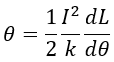# Why Scale of Moving Iron Instrument is Non-uniform?

## Scale of Moving Iron Instrument :

#### In moving iron instruments, the deflection is given by,Where,I = RMS value of the currentk = Control spring constantdL/dθ = Change of inductance with respect to the deflection

From the above equation, it can be infrared that the deflection is directly proportional to the square of the RMS value of the current and the moving iron instruments exhibit square law response. The shape of the scale of moving iron instruments is non-uniform because the deflection θ is proportional to the square of the current.

When the saturation of the coil is assumed to be zero, the rate of change of inductance with respect to the deflection is constant and the instrument follows a pure square law response due to which the scale of the instrument can be designed easily.

In practical instruments, because of the effect of the saturation, dL/dθ is not constant and it depends on the position in which the iron disc deflects. Hence, in these instruments the scale is distorted and it does not follow pure square law response.

However, the above problem can be eliminated by proper selection of the dimensions of the moving coil, and hence the scale can be made approximately uniform. The deflection in the moving iron instrument θ is given as,

Hence, for obtaining a uniform scale over a certain length, the product of deflection and the change of the inductance with respect to the deflection must be constant. However, it is not possible. The variation of dL/dθ with respect to θ is shown in the figure,

From the figure, the shape of the scale of moving iron instruments is a rectangular hyperbola and it is clear that the scale is cramped or compressed at its lower and higher ends. The scale cannot be expanded near the zero reading since dL/dθ would be infinite if it is expanded which is highly impossible.

The scale is compressed at its lower end because the value of the square of rms operating current is less compared to that of full scale reading and the scale is compressed at its higher end because the rate of change of inductance with respect to the deflection decreases drastically at its higher end. The scale is uniform from maximum deflection θ to 0.1 of θ.

## Scale of Moving Coil (PMMC) Instrument :

#### In moving coil instruments, the deflection is given by,θ = GI/KWhere,G = galvanometer constantK = spring constantI = current flowing through the meter

From the above equation, it is clear that the deflection is directly proportional to the current passing through the meter. Thus, the shape of the scale of PMMC instruments is uniform. The below shows the difference between scales of moving iron and moving coil instruments.

Do not enter any spam links and messages